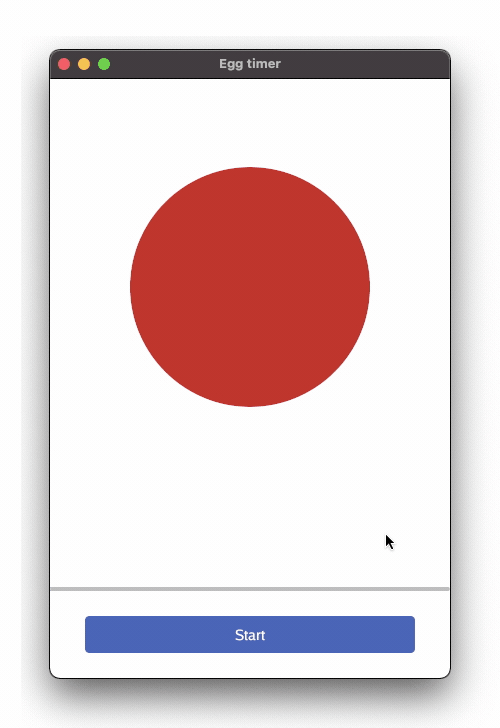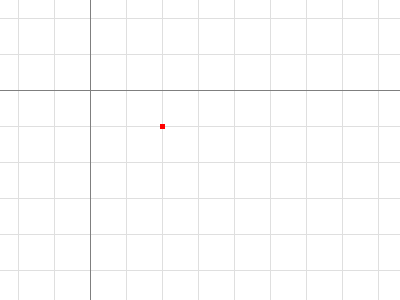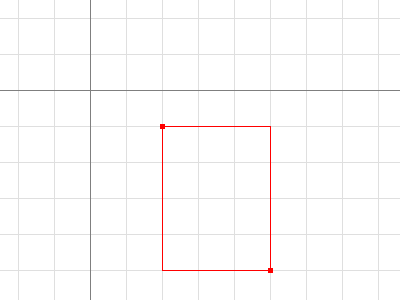# Chapter 8 - Custom graphics - a circle

## Goals

The intent of this section is to draw custom graphics that (vaguely) resembles an egg## Outline

The code introduces custom graphics. The circle in the app is drawn by Gio, not displaying a static picture. Tho achieve that we combine

• A clip to define the area we can draw within
• A paint operation to fill that area
• Some parameters to set color

## Imports

There are some new imports, namely

• image and image/color, Go’s standard 2D image library.

• Nigel Tao writes well about these packages on the Go blog.
• f32. Go’s image library is based on `int`, while Gio for some of its functions works with `float32`. Hence f32 reimplements floating point versions of the two main types, `Points` and `Rectangles`.

• op/clip is used to define an area to paint within. Drawing outside this area is ignored.

• op/paint contains drawing operations to fill a shape with color.

## Points and Rectangles

Points and Rectangles are used extensively, so it’s worth quoting from Nigel’s blog mentioned above. Point’s are coordinates and Rectangles are defined by Points:

``````type Point struct {
X, Y float32
}

type Rectangle struct {
Min, Max Point
}
``````

A Point is an X, Y coordinate pair. The axes increase right and down (origin = top left corner). It is neither a pixel nor a grid square. A Point has no intrinsic width, height or color, but the visualizations below use a small colored square.``````p := image.Point{2, 1}
``````

A Rectangle contains the points with Min.X <= X < Max.X, Min.Y <= Y < Max.Y. It has no intrinsic color, but the visualization below outlines it with a thin colored line, and call out their Min and Max Points.``````r := image.Rect(2, 1, 5, 5)
``````

For convenience, image.Rect(x0, y0, x1, y1) is equivalent to `Rectangle{Point{x0, y0}, Point{x1, y1}}`, but is much easier to type. It also swaps Minimum and Maximum to ensure it’s well formed.

That’s it. Let’s look at the code:

## Code

``````layout.Rigid(
func(gtx C) D {
circle := clip.Ellipse{
// Hard coding the x coordinate. Try resizing the window
Min: image.Pt(80, 0),
Max: image.Pt(320, 240),
// Soft coding the x coordinate. Try resizing the window
//Min: image.Pt(gtx.Constraints.Max.X/2-120, 0),
//Max: image.Pt(gtx.Constraints.Max.X/2+120, 240),
}.Op(gtx.Ops)
color := color.NRGBA{R: 200, A: 255}
paint.FillShape(gtx.Ops, color, circle)
d := image.Point{Y: 400}
return layout.Dimensions{Size: d}
},
),
``````

We first define a circle using `clip.Ellipse{ }`. It defines circle as an `Ellipse` within a box, where the dimensions of the box are specified by the top left and bottom right corners. `Min` and `Max` respectively.
`color.NRGBA` defines the color of the circle. Note that the Alpha-channel defaults to 0, i.e. invisible, so we lift it to 255 so we can actually see it.
`paint.FillShape` fills the shape with the `color`.
And finally we return the widget in the form of its `Dimensions`, height: 400.